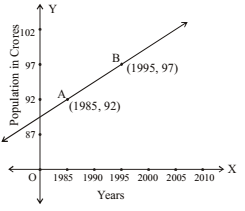## NCERT Solutions for Class 11 Maths Chapter 10 Straight Lines Exercise 10.1

NCERT Solutions of Chapter 10 Straight Lines Exercise 10.1 is given here that are detailed and accurate so you can easily solve difficult questions without wasting your time. NCERT Solutions for Class 11 Maths will increase an individual's problem solving skills and increase his efficiency. These NCERT Solutions will improve your marks and solve complex problems easily.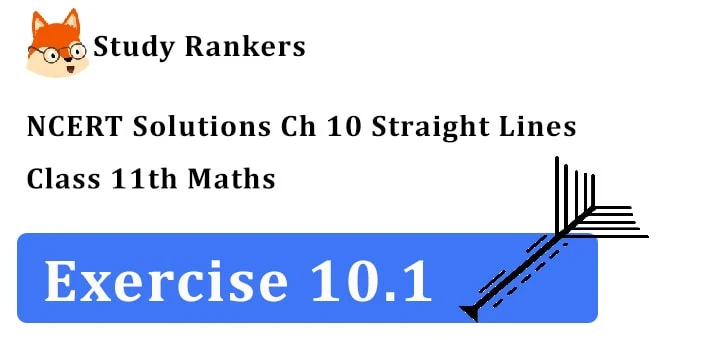1. Draw a quadrilateral in the Cartesian plane, whose vertices are (– 4, 5), (0, 7), (5, –5) and (– 4, – 2). Also find its area.

Given points (– 4, 5), (0, 7), (5, –5) and (– 4, 2) are plotted on a graph paper. They are denoted by A, B, C and D respectively.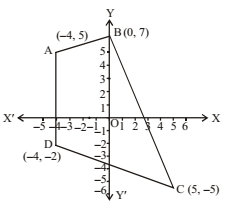Now, divide the quadrilateral into two triangle viz. △ABD and △BDC.
Area of a triangle is
= 1/2 I x1(y2 – y3) + x2(y3 – y1) + x3(y1 – y2)I
The verticals of △ABD are (-4, 5), (0, 7) and (-4, -2)
Area of △ABD
= 1/2 I -4(7 + 2) + 0.(-2 – 5)+ (-4)(5 – 7) I
= 1/2 I -36 + 8 I = 28/2 = 14        ….(i)
The vertices of △BCD are (0, 7), (5, -5), (-4, -2)
Area of △BCD
= 1/2 I 0(-5 + 2) + 5(-2 -7) – 4(7 + 5) I
= 1/2  I -45 – 48 I = 93/2     ……(ii)
= Area of △ABD + area of △BCD
= 14 + 93/2           [from (i) & (ii)]
= (28 + 93)/2 = 121/2 = 60.5 sq. units.

2. The base of an equilateral triangle with side 2a lies along the y-axis such that the mid-point of the base is at the origin. Find vertices of the triangle.

BC is the base of △ABC such that BO = OC = a
AB = AC = 2a
Now, AO2 = AB2 - BO2
∴ AO = √(4a2 – a2) = √3a
∴ The point A lying on x-axis is (√3a, 0)The point B is (0, a) and the point C is (0, – a), when A lies on the left of y-axis the vertices of triangle are (–√3a, 0) a (0, a) and (0, – a).

3. Find the distance between P(x1, y 1) and Q(x2, y 2) when: (i) PQ is parallel to the y-axis, (ii) PQ is parallel to the x-axis.

(i) When PQ is parallel to y-axis, every point on this line has the same abscissa, let
2 = x 1.
The points P and Q are P(x 1, y 1 ),Q(x 2, y 2)
∴ PQ = |y 2 – y1|(ii) When PQ is parallel to x-axis. Every point on the line has the same ordinate.
Let y 2 = y 1
∴ The coordinates of points P and Q are
P(x 1, y 1), Q(x 2, y 2)
∴ PQ = |x 2 – x 1|4. Find a point on the x-axis, which is equidistant from the points (7, 6) and (3, 4).

Let the point on the x-axis be (x 1, 0). The other two points A and B are A(7, 6), B (3, 4)
we have
PA = PB
or PA2 = PB2
or (x 1 – 7)2 + 62 = (x 1 – 3)2 + 42
or x12 – 14x1 + 49 + 36 = x12 – 6x1 + 9 + 16
or –14 x+ 85 = –6x1 + 25
or 8x 1 = 60
or x1 = 60/8
∴ x1 = 15/2
∴ The point on x-axis equidistant from A and B is (15/2 , 0).

5. Find the slope of a line, which passes through the origin, and the mid-point of the line segment joining the points P(0, –4) and B(8, 0).

Coordinates of mid-point of two points (x 1, y 1) and (x 2, y 2) are ((x1 + x2)/2 , (y1 + y2)/2 )
The mid-point of B(8, 0) and P(0, –4) is
((8 + 0)/2 , (0 – 4)/2) i.e., (4, -2)The slope of line joining the points (x 1, y 1) and (x 2, y 2) = (y2 – y1)/(x2 – x1)
The two points are O(0, 0) and A(4, –2)
∴ slope = (-2 – 0)/(4 – 0) = -1/2

6. Without using Pythagoras theorem, show that the points (4, 4), (3, 5) and (–1, –1) are the vertices of a right angled triangle.

We have three points A(4, 4), B(3, 5) and C (–1, –1)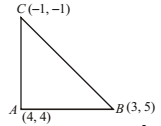∴ Slope of AB = (y2 – y1)/(x2 – x1) = (5 – 4)/(3 – 4) = -1
Slope of AC = (-1 -4)/(-1 – 4) = -5/-5 = 1
Product of slopes of AB and AC = 1× -1 = -1
∴ AB ⊥ AC
Hence, △ABC is a right angled triangle.

7. Find the slope of the line, which makes an angle of 30° with the positive direction of y-axis measured anticlockwise.

The line OP makes an angle of 30° with y-axis measured anti-clock-wise.∴ OP makes an angle of 90 + 30 = 120 with positive direction of x-axis
∴ Slope of OP = tan 120° = tan (180° – 60°)
= –tan 60° = –√3

8. Find the value of x for which the points (x, –1), (2, 1) and (4, 5) are collinear.

We have the points A(x, – 1), B(2, 1), C(4, 5).
A, B, C are collinear if the
Slope of AB = Slope of BC.
Slope of AB = (1 + 1)/(2 – x) = 2/(2 – x);
Slope of BC = (5 – 1)/(4 – 2) = 4/2 = 2
∴ 2/(2 – x) = 2 or 2 – x = 1 or x = 1

9. Without using distance formula, show that points (–2, 1), (4, 0), (3, 3) and (–3, 2) are the vertices of a parallelogram.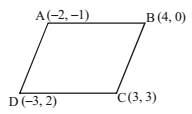The given points are A(–2, –1), B(4, 0), C(3, 3), D(–3, 2)
Slope of AB = 1/(4 + 2) = 1/6;
Slope of DC = (3 – 2)/(3 + 3) = 1/6
∴ Slope of AB = Slope of DC
=> AB || DC
Slope of AD = (2 + 1)/(-3 + 2) = 3/-1;
Slope of BC = (3 – 0)/(3 – 4) = 3/-1
∴ Slope of AD = Slope of BC
=> AD parallel BC Hence, ABCD is a parallelogram.

10. Find the angle between the x-axis and the line joining the points (3, –1) and (4, –2).

Slope of the line joining the point P(3, –1) and Q (4, –2) is equal to (-2 + 1)/(4 – 3) = -1/1 = -1
tan θ = –1 ∴ θ = 135°

11. The slope of a line is double of the slope of another line. If tangent of the angle between them is 1 3, find the slopes of the lines.

Let m1 and m2 be the slopes of the two lines
Given that m1 = 2m2
Let θ be the angle between the line,
tan θ = 1/3 = (m1 – m2)/(1 +m1m2)
putting m1 = 2m2, 1/3 = (2m2 – m2)/(1 + 2m2m2) = m2/(1 + 2m22)
or 3m2 = 1 + 2m22 or 2m22 – 3m2 + 1 = 0
or (2m2 – 1)(m2 -1) = 0 m2 = 1 or 1/2
since, m1 = 2m2
∴ for m2 = 1, m1 = 2, for m2 = 1/2, m1 = 1
∴ slopes of these lines are 1, 1/2 or 2, 1

12. A line passes through (x1, y 1) and (h, k). If slope of the line is m, show that
k – y 1 = m(h – x1).

Slope of the line joining the points A(x 1, y 1) and B(h, k)
= (k – y1)/(h – x1) = m (Given)
Cross-multiplying, k – y 1 = m(h – x 1) Hence proved.

13. If three points (h, 0), (a, b) and (0, k) lies on a line, show that a/h + b/k = 1.

The given points are A(h, 0), B(a, b), C(0, k), they lie on the same plane.
∴ Slope of AB = Slope of BC
∴ Slope of AB = (b – 0)/(a – h) = b/(a – h);
Slope of BC = (k – b)/(0 – a) = (k – b)/-a
∴ b/(a – h) = (k – b)/ -a or by cross-multiplication
–ab = (a – h)(k – b)
or –ab = ak – ab – hk + hb
or 0 = ak – hk + hb
or ak + hb = hk
Dividing by hk => ak/hk + hb/hk = 1 or a/h + b/k = 1
Hence proved.

14. Consider the following population and year graph, find the slope of the line AB and using it, find what will be the population in the year 2010?